Courses

# Test: Chemistry- 1

## 45 Questions MCQ Test NEET Mock Test Series | Test: Chemistry- 1

Description
This mock test of Test: Chemistry- 1 for NEET helps you for every NEET entrance exam. This contains 45 Multiple Choice Questions for NEET Test: Chemistry- 1 (mcq) to study with solutions a complete question bank. The solved questions answers in this Test: Chemistry- 1 quiz give you a good mix of easy questions and tough questions. NEET students definitely take this Test: Chemistry- 1 exercise for a better result in the exam. You can find other Test: Chemistry- 1 extra questions, long questions & short questions for NEET on EduRev as well by searching above.
QUESTION: 1

### Two oxides of metal contain 27.6℅ and 30℅ oxygen respectively. If formula of first oxide is M3O4, then formula of second oxide is:

Solution:

Given that, formula of first oxide = M3O4
Let mass of the metal = x
% of metal in M3O4 = (3x / 3x + 64) * 100
but as given % age = (100 - 27.6) = 72.4%
so, (3x / 3x + 64) * 100 = 72.4
or x = 56.
in 2nd oxide,
oxygen = 30%....so metal = 70%
so, the ratio is
M : O
70 / 56 : 30 / 16
1.25 : 1.875
2 : 3
so, 2nd oxide is M2O3

QUESTION: 2

### 5.6 litres of a gas at N.T.P. are found to have a mass of 11 g. The molecular mass of the gas is

Solution:

5.6lit = 11g
22.4lit = ? (x) g
x = 22.4 × 11 / 5.6
x = 44g
Thus, Molecular mass of gas is 44g

QUESTION: 3

### The crystalline salt, Na2SO4xH2O on heating loses 55.9% of its weight. The formula of the crystalline salt is

Solution:

Molar mass of salt = (2 × 23) + 32 + (16 × 4) + x × 18
= 142 + 18x
On heating it will lose water and become anhydrous. 55.9% mass is the mass of water in Na2SO4.xH2O.
Mass by mass% = (mass / molar mass of compound) × 100
mass of water = 18x
55.9 = (18x / 142 + 18x) × 100
55.9(142 + 18x) = 1800x
7937.8 + 1006.2x = 1800x
x = 9.99
x = 10 (approx.)
So, the molecular formula of compound is Na2SO4.10H2O.

QUESTION: 4

0.5 g of a metal on oxidation gave 0.79 g of its oxide. The equivalent weight of the metal is (approximately)

Solution:

Given,

Mass of metal = 0.5 g

Mass of oxide = 0.79 g

First we have to calculate the Mass of Oxygen in metal oxide.

Mass of Oxygen = Mass of oxide - Mass of Metal = 0.79 - 0.5 = 0.29 g

The equivalent weight of oxygen is 8 g/eq.

0.29 g of oxygen combines with the 0.5 g of metal

8 g of oxygen combines with  of metal.

Therefore, the equivalent weight of metal is 13.793 g/eq.

QUESTION: 5

Air contains nearly 20% oxygen by volume. The volume of air needed for complete combustion of 100 cc of acetylene will be

Solution:

Equation
2 C2H2 + 5O2 → 4CO2 + 2 H2O
In this reaction, we can see that, 2 moles of C2H2reacts with 5 moles of O2​
Or, 1 mole of ​C2H2 reacts with 5 / 2 moles of O2​
Under standard conditions, we can say that:
22.44 L of C2H2 reacts with (5 / 2) x 22.4 L moles of O2
So, 100 cc of C2H2 will reacts with (5 / 2) x 100 cc = 250 cc of O2​ .
But, since air contains 20 % of oxygen by volume, the amount of air needed to react with 100 cc of C2H2 will be = 250 x (100 / 20) = 1250 cc of air.

QUESTION: 6

A solution of 10 ml(M/10) FeSO4 was titrated with KMnO4 solution in acidic medium. The amount of  KMnO4 used will be

Solution:

The equation of the Mn2+ in acidic medium  will be.

MnO4- + 8H++ 5 Fe2+ → Mn2++ 5Fe3+ +4H2O.

We can balance the reaction by using redox balancing of acid and base.

So, we know from the equation that 5 moles of iron react to form 1 mole of Mn+2.

Thus, we can say that (10*0.1) moles of iron will react with the (10*0.1/5) moles of Manganese ions, which will be 0.2 thus, 10ml volume of KMnO4 and 0.02M moles of KMnO4.  We will get the same result if we use M1V1 = M2V2 formulae.

QUESTION: 7

At S.T.P. the density of CCl4 vapour in g/L will be nearest to

Solution:

Molecular Weight of CCl₄ = 35.5 + 12 x 4 = 154
1 mole CCl₄ vapour=154 g
According to Avogadro’s hypothesis we know that the molar volume of a gas at STP is 22.4 litres
= 154 / 22.4 = 6.87

QUESTION: 8

The law of multiple proportions was proposed by

Solution:

Dalton's law of multiple proportions is part of the basis for modern atomic theory, along with Joseph Proust's law of definite composition (which states that compounds are formed by defined mass ratios of reacting elements) and the law of conservation of mass that was proposed by Antoine Lavoisier.

QUESTION: 9

The anode rays were discovered by

Solution:

The discovery of protons can be attributed to Rutherford. In 1886 Goldstein discovered existence of positively charged rays in the discharge tube by using perforated cathode. These rays were named as anode rays or cannal rays.

QUESTION: 10

Which of the following transitions of electrons in the hydrogen, atom will emit maximum energy ?

Solution:

Energy is given by formula
E = hc / λ
1 / λ = RZ2 [ 1 / n21 - 1 / n22 ]
E = RZ2 hc [ 1 / n21 - 1 / n22]
This energy will be highest when the transition is from n3 → n2.

QUESTION: 11

The uncertainity found from the uncertainity principle (Δx.Δp = h / 4π) is

Solution:

Δx.Δp ≧ h / 4π
So by the equation given in the question, the uncertainity value will be the least.

QUESTION: 12

The number of orbitals in any shell (n) is equal to

Solution:

Maximum number of electrons can be accommodated in a shell of principal quantum number n is 2n2. Since each orbital can accommodate only 2 electrons, maximum number of orbitals =2n2/2= n2

QUESTION: 13

The maximum number of 4f electrons having spin quantum number (-1/2) is

Solution:

Total no. of electrons in F- subshell = 14 (when we include both spin +1/2  & - 1/2)
But maximum no. of electrons = 7 if only one spin either - 1/2 or +1/2.

QUESTION: 14

The number of unpaired electrons in Cu+  (Z= 29) is

Solution:

Answer : The electronic configuration of Cu is,

1s22s22p63s23p64s13d10

The reason for abnormal electronic configuration of Cu is because of the half-filled and fully-filled configuration are the more stable configuration. In this configuration, the d-subshell is fully-filled and s-subshell is half-filled which makes the Cu more stable.

Now, for getting a positive charge, it has to lose 1 electron which will be removed from the outermost 4s orbital and hence all the remaining electrons will be paired leading to 0 unpaired electrons.

QUESTION: 15

The electrons present in K-shell of the atom will differ in

Solution:

Two electrons in K-shell will differ in spin quantum number.
They will have same values for the principal quantum number, azimuthal quantum number, and magnetic quantum number.

For first electron, n = 1,l = 0,m = 0,s = +1 ​/ 2
For second electron, n = 1,l = 0,m = 0,s = −1 / 2​

QUESTION: 16

Consider the following statements. The d-orbitals have

I. four lobes and two nodes

II. four lobes and one node

Which of the above statements are correct ?

Solution:

Total no of angular nodes = l (azimuthal quantum no), so l=2 in d orbital, therefore number of nodes is 2 and it is a fact that opposite lobes have same signs and adjacent ones have opposite signs. there are 4 lobes in d orbital.

QUESTION: 17

Which of the following substances has the lowest boiling point?

Solution:

Boiling Point is directly proportional to VanderWaals forces of attraction. Down the group, this forces increases. so force of attraction in CHF3 is weaker than CHL3, that's why boiling point of CHF3 is lowest.

QUESTION: 18

Which of the following is solid with highest melting point ?

Solution:

SiO2 is a covalent 'network' solid. So the energy required to break bonds will be higher. Therefore the melting point will be higher.

QUESTION: 19

Out of the two compounds shown below, the vapour pressure of B at a particular temperature is expected to be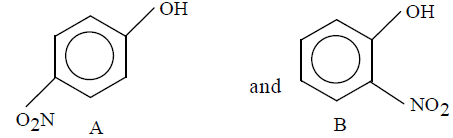Solution:

Due to intramolecular H-bonding  in o-nitrophenol it exists as a monomer while due to intermolecular H-bonding in p-nitrophenol it exists as an associate molecule. As a result, vapour pressure of a o-nitrophenol is higher than that of a p-nitrophenol.

QUESTION: 20

In which of the following molecules, the central atom does not have sp3 hybridisation?

Solution:

When the number of hybrid orbitals, H  is 4, the hybridization is sp3 hybridization
H = 1 / 2[V + M - C + A]
where, V = number of monovalent atoms
C = total positive charge
A = negative charge
a) For CH4
H = 1 / 2[4 + 4 - 0 + 0] = 4
sp3
b) For SF4,
H = 1 / 2 [6 + 4 - 0 + 0] = 5
sp3d
c) For BF-4,
H = 1 / 2 [3 + 4 - 0 + 1] = 4, thus sp3
d) For NH+4
H = 1 / 2[5 + 4 - 1 + 0] = 4 thus sp3
Thus, only in SF4 the central atom does not have sp3 hybridisation.

QUESTION: 21

The correct oreder of increasing bond angles in the following species

Solution:

According to VESPR theory repulsion order

lp - lp > lp - bp > bp - bp

As the number of lone pairs of electrons increases, bond angle decreases due to repulsion between lp-lp, Moreover, as the electronegativity of central atom decreases, bond angle decreases.
The correct order of increasing bond angle is Cl2O < ClO2- < ClO2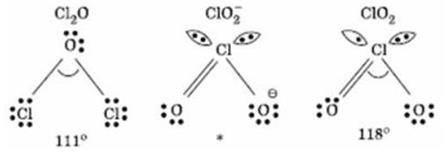In ClO2- there are 2 Ione pairs of electrons present on the central chlorine atom. Therefore the bond angle in ClO2is less than 118° which is the bond angle in ClO2 which has less number of electrons on chlorine.

QUESTION: 22

The correct order of increasing covalent character of the following is

Solution:

Option b is correct  because as the charge increases covalent character also increases.

QUESTION: 23

If Z-axis is the molecular axis, then π molecular orbitals are formed by the overlap of

Solution:

px-px and py-py will form pi bond when z-axis is molecular axis as the options have only one of these so option (d) is the correct answer.

QUESTION: 24

The ionic species having largest size is

Solution:

Li+ have larger size in aq.solution due to the presence of the water molecules present inside the solution surronds easily and become the solution to be more hydrated comparing to the corresponding element present in the aqeous solution.

QUESTION: 25

From the following species, which one is the easiest to remove one electron ?

Solution:

Ionisation Potential is directly proportional to positive oxidation state and inversely proportional to negative oxidation state.So, in O2- removal of electron is easiest.

QUESTION: 26

Electron gain enthalpy of inert gases is

Solution:

On moving down the group, there is an increase in the size of atoms. As a result, the tendency to accept an additional electron decreases and hence, the electron gain enthalpy decreases from Ne to Rn. However, He has small size and much higher tendency to accept an additional electron. Hence, its electron gain enthalpy is least positive of all the noble gases.

Thus, it is concluded that neon has the highest positive electrons gain enthalpy.

QUESTION: 27

The electron gain enthalpies of B,C,N and O are in the order

Solution:

Actually electron gain enthalphy and ionisation energy both are dependent on electronic configuration.
Electronegativity means tendency to attract electron it doesnot matter where it will keep the electron
Electron gain entalphy basically refer to attract e it must have space to keep electron in sub shell like spdf should be present
order of electronegativity is
F > O > N > Cl > Br  > I > S > C > P

QUESTION: 28

With respect to chlorine, hydrogen will be

Solution:

With respect to Chlorine hydrogen will be electropositive. Chlorine being a strong electronegative element tends to force hydrogen to loss electron and hydrogen acquire electropositive character so that chlorine can complete its octet .

QUESTION: 29

Which element has the greatest tendency to lose electrons ?

Solution:

Francium has the greatest tendency to lose electrons.

QUESTION: 30

Which of the following atomic numbers represents an alkali metals ?

Solution:

37 = Rb
55 = Cs
87 = Fr
All belong to alkali metal group.

QUESTION: 31

LiNO3 on heating gives

Solution:

Lithium nitrate when heated decomposes to give lithium oxide and nitrogen dioxide. The reaction is as follows :

4LiNO₃ → 2Li₂O+ 4NO₂+ O₂

LiNO₃ on heating, gives reddish brown fumes of NO₂

QUESTION: 32

Which of the following is an ore of potassium ?

Solution:

Carnallite is an ore of potassium i.e., KCl.MgCl2.6H2O.

QUESTION: 33

Which of the following decomposes at highest temperature ?

Solution:

BaCO3 decomposes at highest temp.

All the carbonates decompose on heating to give CO2 and metal oxide.The stability of carbonate towards heat depends upon the stability of the resulting metal oxide. More is the stability of the resulting metal oxide lesser is the stability of the carbonate towards heat and vice versa.

QUESTION: 34

Nitrolim which is used as a fertilizer has the composition

Solution:

Calcium cyanamide (CaCN2) mixed with carbon (C) is called nitrolim.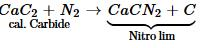QUESTION: 35

The difference of water molecules in gypsum and plaster of paris is

Solution:

Gypsum is CaSO4.2H2O and Plaster of Paris is CaSO4.1 / 2H2O. Difference in number of water molecules = 3 / 2 or 1 x 1 / 2

QUESTION: 36

Which of the following does not contain fused benzene rings ?

Solution:

In Diphenyl the two Benzene rings share a carbon -carbon bond but not the two carbon atoms.

QUESTION: 37

The IUPAC name of the compound,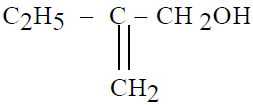is

Solution:

As the double bond has to be taken in main chain and also the carbon to with functional group has to be numbered first thus option A is the correct one.

QUESTION: 38

IPUAC name of the compound,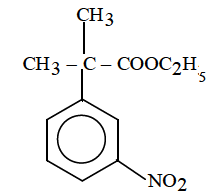Solution:

The IUPAC name of the given compound  is Ethyl-2-methyl-2-(3-nitrophenyl) propanoate,because the side chain contains the functional group , then the phenyl ring is considered as a substituent.

QUESTION: 39

The total number of structural isomers (acyclic and cyclic) possible for the molecular formula, C6H6 are

Solution:

1 linear and 1 cyclic benzene ring.

QUESTION: 40

Which of the following does not show tautomerism ?

Solution:

For an aldehyde or a ketone to exhibit tautomerism it is essential that it must have at least one α−Hα−H atom.

So, C6H5COC(CH3)3

Hence D is the correct answer.

QUESTION: 41

The number of geometrical isomers in case of compound CH3–CH=CH–CH=CH–C2H5 are

Solution:

Two pairs of cis and trans forms.

QUESTION: 42

The most stable free radical among the following is

Solution:

The most stable free radical is C6H5CHCH3 because of resonance.

QUESTION: 43

Which of the following is correct regarding the - I effect of the substituents ?

Solution:

The electronegativity of F, O, and N follows the order: N < O < F

Therefore the negative inductive effect of - NR2, -OR and -F follows the order:

-NR2 < -OR < - F

QUESTION: 44

Bond length of ethane (I), ethene (II), acetylene (III) and benzene (IV) follows the order

Solution:

Bond Length ∝ 1 / Bond Order

So, the correct order of bond length is

I > IV > II > III

QUESTION: 45

Which of the following is the strongest nucleophile?

Solution:

Methyl grps are electron rich, donate electrons and create a +I effect, and
NUCLEOPHILE means a electron rich atom